Jonathan Sondow (1943 - 2020)A parabolic analog of the arbelos, fromBefore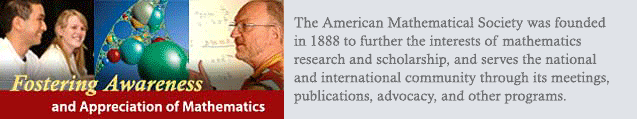Giving a talk at a meeting of theAfter

This memorial page is a modified version of Jonathan Sondow's personal home page which may or may not still be available.

 EDUCATION: Ph.D. 1965 Princeton University Advisor: B.A.-with-Honors 1962 Diploma and Calculus Prize 1959

 MILNOR WINS THE 2004 STEELE PRIZE FOR EXPOSITION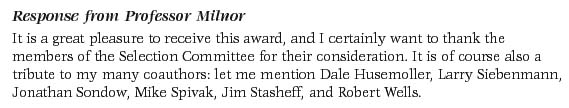FOOTNOTE TO MILNOR'S "DIFFERENTIAL TOPOLOGY 46..."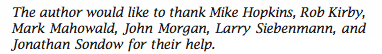DANI WISE'S RESPONSE TO WINNING THE VEBLEN PRIZE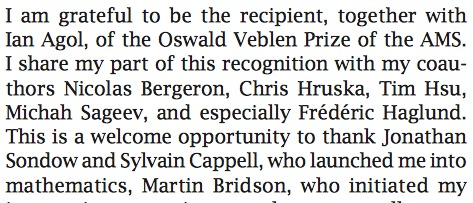NEW AND NOTEWORTHY 18 December 2018

 * arXiv versions of [63, 74], Zbl reviews of [63, 70], online version of , new papers [38, 69, 72, 73, 74]

 * Designation of  as the 6th most-cited Monthly paper on pi in [xlxvi]'s list of ``pi-star'' papers

 * Co-author links in  to a tribute page for, and in  to a paper by, my friend and coauthor Sylvester Reese, who passed away 21 May 2014

 * Link in  to Hajja's Zentralblatt review: "The paper under review is a major contribution to the literature on the arbelos. For the first time, someone dares make drastic changes on the Archimedean configuration..."

 * Link in [xxxv] to Paul Loya's book "Amazing and Aesthetic Aspects of Analysis." My symmetric formula for pi (see below) is a "beautiful expression" and "quite astonishing!"

 * Belated publication of my solution  to my Monthly Problem 11222: An Infinite Product Based on a Base; the editor comments "The proposer's elegant solution covers all cases simultaneously and efficiently."

 * Link [xxvi] to Michel Waldschmidt's Bombay lectures on irrationality; he mentions my work on Euler's constant  and gives my geometric proof that e is irrational and my irrationality measure for e 

 * At long last, the MAA has put a citation of  in Dunham's book "Euler, the Master of Us All", and PUP has cited  in Havil's book "Gamma: Exploring Euler's Constant" - see [I] and [III] below

 * Link [xviii] to John Baez's web page This Week's Finds in Mathematical Physics. He displays the infinite products for e, e^gamma, and pi/2 shown below, calls them "eerily similar" and a "mystery thrown down to us by the math gods, like a bone from on high", and cites my Monthly note "A faster product for pi ..." .

 * Link [VII] to A. V. Zhukov's book El Omnipresente Número "Pi". My symmetric formula for pi (see below) is an exhibit in his "museum of elegant mathematics."

FORMULAS AND PLOTS FROM MY WORK
(SCROLL DOWN FOR CITATIONS AND LINKS TO PAPERS)

 Conjectured by Knopp and first proved by Hasse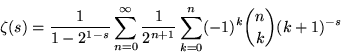A global series rediscovered in , ; cited in [iii], [viii], [III], [IV], [VIII], [IX]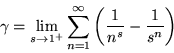Antisymmetric formula for Euler's constant , cited in [i], [iv], [I], [II], [III], [VI]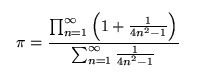Symmetric formula for pi , cited in [vi], [xxxv], [VII]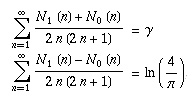Series with the number of 1s and 0s in the binary expansion of n, from , , cited in [xxxii]

The product below for e is due to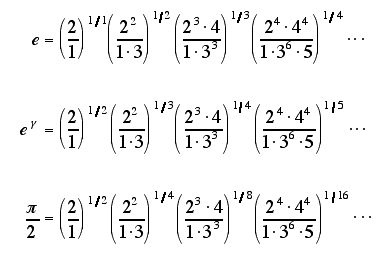Infinite products from , , cited in [iv], [xviii], [xxiii]; the 2nd found earlier by J. Ser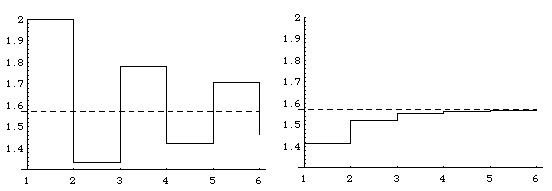Partial products of Wallis's product (left) and my faster product for pi/2, from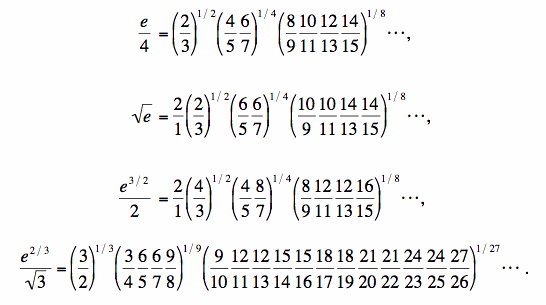Catalan-type infinite products, from  with Yi HUANG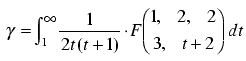Hypergeometric formula for Euler's constant ,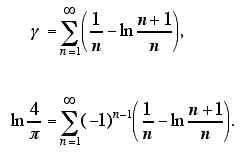Series showing ln(4/pi) is an "alternating Euler constant" , , , cited in [iv], [xxxii]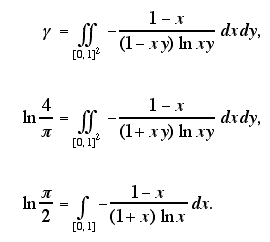Integrals from , , cited in [i], [iv], [XI]. The 3rd is a special case of Kummer's integral.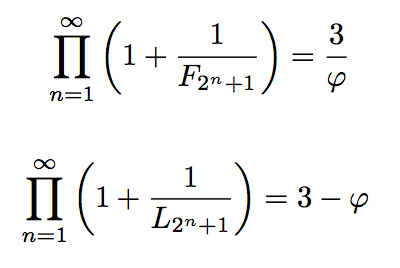Fibonacci- & Lucas-number products from , where phi is the golden ratio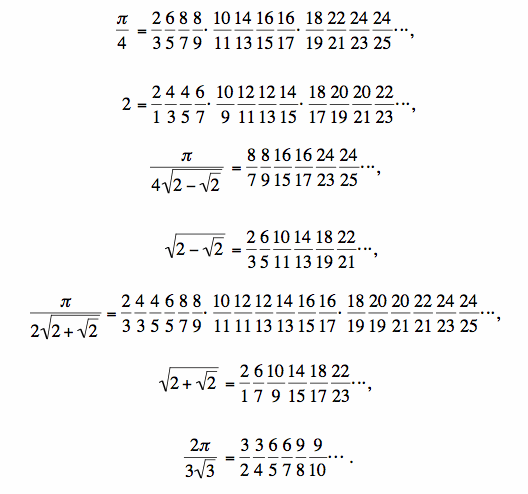Wallis-type infinite products, from  with Yi HUANG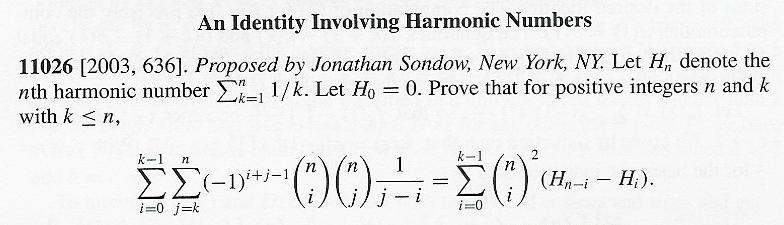Monthly Problem: a new binomial coefficient identity ,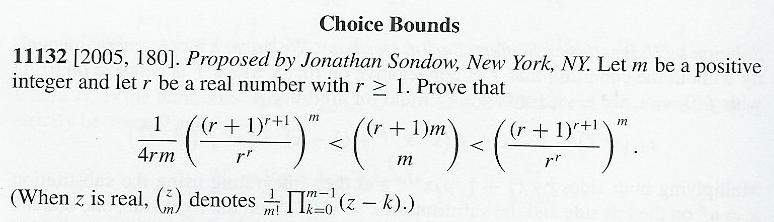Monthly Problem: inequalities for generalized binomial coefficients, from  and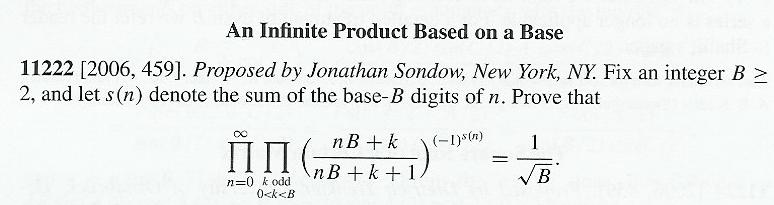Monthly Problem: a new base-B generalization of the Woods-Robbins product (B=2), from  and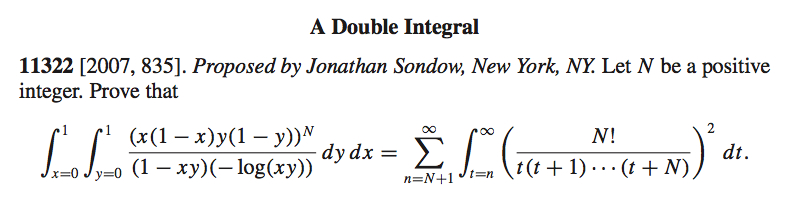Monthly Problem: Beukers-type double integral = Nesterenko-type series , , , , [VI]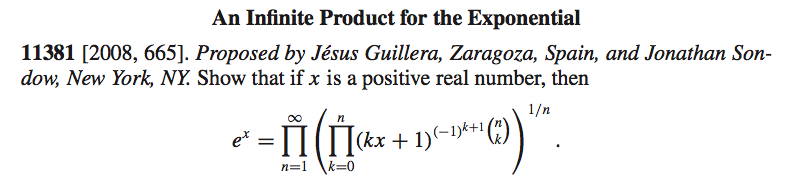Monthly Problem: An infinite product for e^x , from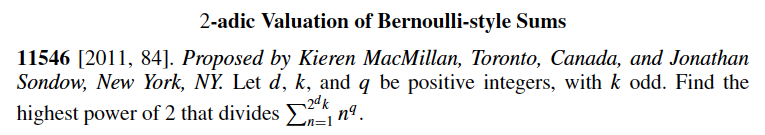Monthly Problem: the highest power of 2 dividing a power sum , from , cited in [xxxiii]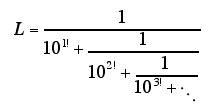The continued fraction L is a Liouville number with irrationality base one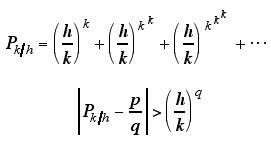A number with irrationality base k/h > 1, and an inequality for all integers p,q with q large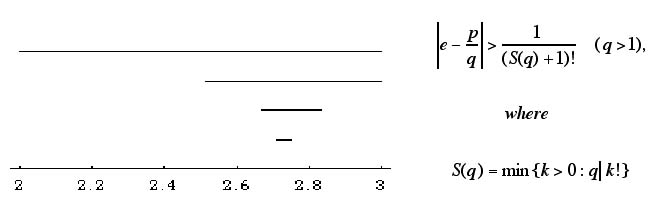A geometric proof that e is irrational, and a new measure of its irrationality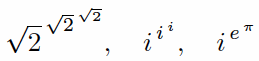Transcendental numbers if Schanuel's Conjecture holds, from  with Diego MARQUES, cited in [xlii]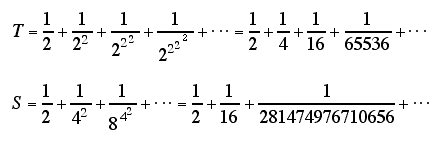T has irrationality base 2 and S has irrationality base infinity , 

FOR THE THREE PLOTS BELOW I THANK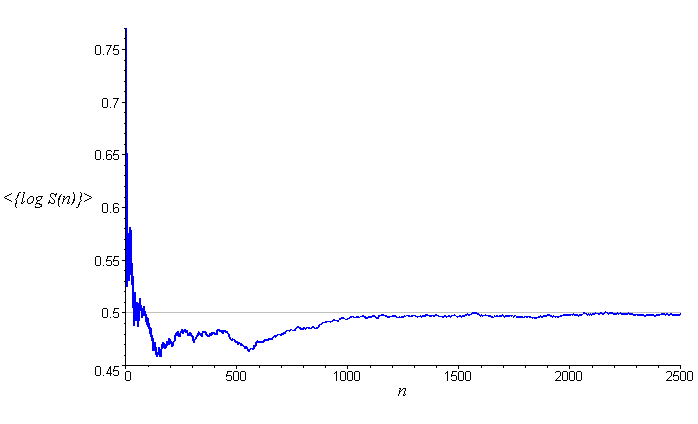If <{log S(n)}> does NOT tend to zero as n increases, then Euler's constant is irrational ,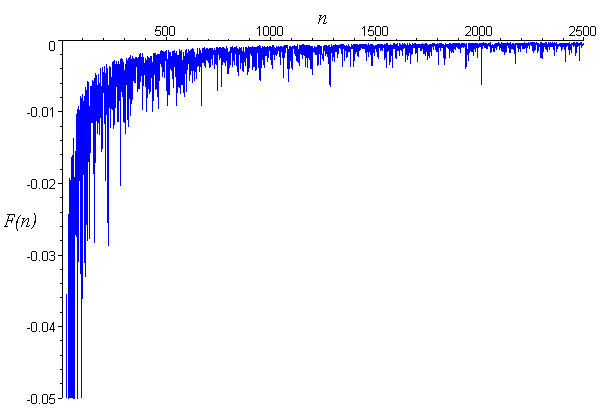If F(n) -> 0, then Euler's constant is irrational, but is not a super Liouville number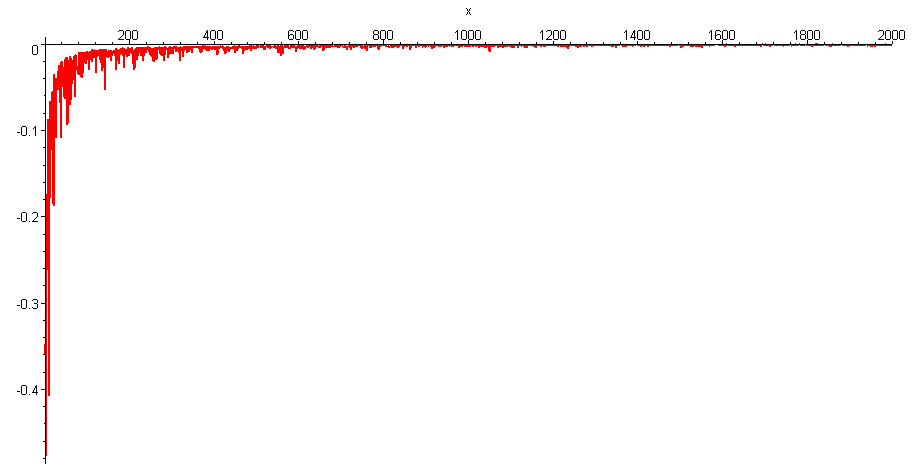If the graph -> 0 as n increases, then ln(pi) is irrational, but isn't a super Liouville number 

SOME WEB PAGES AND ARTICLES CITING MY WORK IN NUMBER THEORY AND GEOMETRY

i. X. Gourdon and P. Sebah's

ii. Matthew Watkins'

iii. Eric Weisstein's
iv. _____________
v. _____________
vi. _____________
vii. ____________
viii. Philippe Biane, Jim Pitman and Marc Yor's
x. Michel Waldschmidt's
xi. American Scientist's
xii. Keith Matthews'
xiii. ____________
xiv. Neil J. Sloane's
xv. ____________
xvi. ___________
xvii. ___________
xviii. John Baez's
xix. Stefan Kraemer's
xx. Michel Waldschmidt's
xxi. _______________
xxii. _______________
xxiii. Jesus Guillera's
xxiv. Michel Waldschmidt's
xxv. _______________
xxvi. _______________
xxvii Wikipedia's
xxviii. _________
xxix. Benoît Rittaud's
xxx. Nuit Blanche's
xxxi. Thomas and Joseph Dence's

xxxii. Jean-Paul Allouche's

xxxiii. Doron Zeilberger's

xxxiv. Peter Hegarty's

xxxv. Paul Loya's

xxxvi. PlanetMath's

xxxvii. Yuri Matiyasevich, Filip Saidak, and Peter Zvengrowski's

xxxviii. Robert P. Schneider's
xxxix. Aliza Steurer and Thomas Hagedorn's
xl. Keith Matthews'
xli. Jeffrey Lagarias'
xlii. Steven Finch's
xliii. Emmanuel Tsukerman's
xliv. B. Berndt, S. Kim, and A. Zaharescu's
xlv. Jenda Vondra's
xlvi. Hamza Khelif's
xlvii. Antonio M. Oller-Marcén's
xlviii. Carlos Rivera's
xlix. I. Ben-Ari, D. Hay, and A. Roitershtein's
xlx. Michel Waldschmidt's
xlxi. Wikipedia's
xlxii. Yuri Matiyasevich's
xlxiii. B. Berndt, S. Kim, and A. Zaharescu's
xlxiv. B. Berndt, S. Kim,  M. Phaovibul, and A. Zaharescu's
xlxv. Wolfgang Göbels'
xlxvi. J. M. Borwein and S. T. Chapman's
xlxvii. Jacques Gelinas's
xlxviii. Jacques Gelinas's
xlxix. Presh Talwalkar's
xlxx. Presh Talwalkar's

SOME BOOKS CITING MY WORK IN NUMBER THEORY

 I. by William Dunham, MAA, 1999 (8th printing, 2010), p. 36 (my antisymmetric formula for Euler's constant exhibits "a delightful symmetry")

 II. by Steven Finch, Camb. Univ. Press, 2003, pp. 35, 37

 III. by Julian Havil, Princeton Univ. Press, 2009, pp. 109, 257

 IV. by Eric Weisstein, 2nd ed., CRC Press, 2002, p. 2562

 V. Book of the Year 1967, p. 503

 VI. by George Boros and Victor Moll, Cambridge Univ. Press, 2004, pp. 234, 293-4, 300, 306

 VII. by A. V. Zhukov, Serie de Divulgación Científica Matemática, N11, Moscú : URSS, 2005, p. 159

 VIII. by Jacob Korevaar, Springer, 2004, p. 326

 IX. by Henri Cohen, Springer, 2007, pp. 140, 260

 X. by Hongwei Chen, Math. Assoc. of America, 2010, pp. 252

 XI. by H. M. Srivastava and J. Choi, Elsevier, 2012, pp. 15 (my double integral for Euler's constant is "elegant"), 239, 241, 648

 XII. by Victor Moll, American Math. Society, 2012, pp. xxii, 278, 371, 453-4, 485, 488-9, 501

 XIII. by George Andrews and Bruce Berndt, Springer, 2013, pp. 157, 164, 424, 433

 XIV. by Jonathan Borwein, Alf van der Poorten, Jeffrey Shallit, Wadim Zudilin, CUP, 2014, pp. 159, 205

 MY WORK IN NUMBER THEORY AND GEOMETRY NOTE: links to MR reviews go to PDFs and do NOT require a subscription to MathSciNet

 1. Analytic continuation of Riemann's zeta function and values at negative integers via Euler's transformation of series,Proc. Amer. Math. Soc. 120 (1994) 421-424.

 2. The Riemann Hypothesis, simple zeros, and the asymptotic convergence degree of improper Riemann sums,Proc. Amer. Math. Soc. 126 (1998) 1311-1314.

 3. An antisymmetric formula for Euler's constant, Math. Mag. 71 (1998) 219-220.

 4. Zeros of the alternating zeta function on the line R(s)=1, Amer. Math. Monthly 110 (2003) 435-437.Chinese translation published in: Mathematical Advance in Translation, Chinese Academy of Sciences, 33 #2 (2014) 188-189.

 5. Criteria for irrationality of Euler's constant, Proc. Amer. Math. Soc. 131 (2003) 3335-3344.

 6. Double integrals for Euler's constant and ln 4/pi and an analog of Hadjicostas's formula,Amer. Math. Monthly 112 (2005) 61-65.

 7. Formulas for pi(x) and the nth prime, with . . .

 8. A hypergeometric approach, via linear forms involving logarithms, to criteria for irrationality of Euler's constant, Math. Slovaca 59 (2009) 1-8, with an Appendix by . . .

 9. Euler's constant, q-logarithms, and formulas of Ramanujan and Gosper,Ramanujan J. 12 (2006) 225-244, with . . .

 10. An irrationality measure for Liouville numbers and conditional measures for Euler's constant (2003, e-print).

 11. An infinite product for e^gamma via hypergeometric formulas for Euler's constant, gamma (2003, e-print).

 12. A faster product for pi and a new integral for ln pi/2,Amer. Math. Monthly 112 (2005) 729-734 (article), 113 (2006) 670 (addendum).

 13. Irrationality measures, irrationality bases, and a theorem of Jarnik (2004, e-print).

 14. A geometric proof that e is irrational and a new measure of its irrationality,Amer. Math. Monthly 113 (2006) 637-641 (article), 114 (2007) 659 (addendum).

 15. Corrigendum: On the irrationality of some alternating series,Studia Univ. Babes-Bolyai Math. 49 no. 1 (2004) 105-106, with . . .

 16. Double integrals and infinite products for some classical constants via analytic continuations of Lerch's transcendent, Ramanujan J. 16 (2008) 247-270, with . . .

 17. New Vacca-type rational series for Euler's constant and its "alternating" analog ln 4/pi,

 18. Summation of series defined by counting blocks of digits,J. Number Theory 123 (2007) 133-143, with . . .

 19. Problem 11026 (see image above): An identity involving harmonic numbers,Amer. Math. Monthly 110 (2003) 636 (proposal), 112 (2005) 367-369 (solution).

 20. Problem 11132 (see image above): Choice bounds, Amer. Math. Monthly 112 (2005) 180 (proposal), 114 (2007) 359-360 (solution).

 21. Problem 11222 (see image above): An infinite product based on a base,Amer. Math. Monthly 113 (2006) 459 (proposal), 115 (2008) 954-955 (solution).

 22. Problem 88 (see image above): A symmetric formula for pi, Math Horizons 5 (Sept., 1997) 32, 34.

 23. The generalized-Euler-constant function gamma(z) and a generalization of Somos's quadratic recurrence constant, J. Math. Anal. Appl. 332 (2007) 292-314, with . . .

 24. The Taylor series for e and the primes 2, 5, 13, 37, 463: a surprising connection (2006, e-print).

 25. Integrals over polytopes, multiple zeta values and polylogarithms, and Euler's constant,Math. Notes (in English) 84 (2008) pp. 568-583, Erratum p. 887;Mat. Zametki (in Russian) 84:4 (2008) pp. 609-626; with . . .

 26. A simple counterexample to Havil's "reformulation" of the Riemann Hypothesis,Elemente der Mathematik 67 (2012) 61-67.

 27. Which partial sums of the Taylor series for e are convergents to e? (and a link to the primes 2, 5, 13, 37, 463). Part II (includes corrected version of Part I), with . . .

 28. Infinite products with strongly B-multiplicative exponents,Annales Univ. Sci. Budapest, Sect. Comp. 28 (2008) 35-53 (article), 32 (2010) 253 (errata), with . . .

 29. Primes, pi, and irrationality measure (2007, e-print).

 30. Problem 11322 (see image above): A double integral, Amer. Math. Monthly 114 (2007) 835 (proposal), 116 (2009) 650 (solution).

 31. Problem 11381 (see image above): An infinite product for the exponential,Amer. Math. Monthly, 115 (2008) 665 (proposal), 117 (2010) 283-284 (solution), with . . .

 32. Ramanujan primes and Bertrand's postulate,Amer. Math. Monthly 116 (2009) 630-635.

 33. Reducing the Erdos-Moser equation 1^n + 2^n + … + k^n = (k+1)^n modulo k and k^2,Integers 11 (2011) article A34, with . . .

 34. A monotonicity property of Riemann's xi function and a reformulation of the Riemann Hypothesis,Periodica Mathematica Hungarica 60 (2010) 37-40, with . . .

 35. Algebraic and transcendental solutions of some exponential equations,Annales Mathematicae et Informaticae 37 (2010) 151-164, with . . .

 36. How not to prove/disprove the Riemann Hypothesis (in preparation), with . . .

 37. New Wallis- and Catalan-type infinite products for pi, e, and sqrt(2+sqrt(2)),Amer. Math. Monthly 117 (2010) 912-917, with . . .

 38. Initial sums of the Legendre symbol: Is min + max ≥ 0 ?, with . . .

 39. Lerch quotients, Lerch primes, Fermat-Wilson quotients, and the Wieferich-non-Wilson primes 2, 3, 14771,

 40. Schanuel's conjecture and algebraic powers z^w and w^z with z and w transcendental,East-West Journal of Mathematics 12, no. 1 (2010) 75-84, with . . .

 41. Proofs of power sum and binomial coefficient congruences via Pascal's identity,Amer. Math. Monthly 118 (2011) 549-551, with . . .

 42. Divisibility of power sums and the generalized Erdos-Moser equation,Elemente der Mathematik 67 (2012) 182–186, with . . .

 43. Ramanujan primes: bounds, runs, twins, and gaps,J. Integer Seq. 14 (2011) article 11.6.2, with . . .

 44. Robin's theorem, primes, and a new elementary reformulation of the Riemann Hypothesis, Integers 11 (2011) article A33, with . . .

 45. The Schanuel subset conjecture implies Gelfond's power tower conjecture (2012, e-print), with . . .

 46. Problem 11546 (see image above): 2-adic Valuation of Bernoulli-style Sums,Amer. Math. Monthly 118 (2011) 84 (proposal), 119 (2012) 886-887 (solution), with . . .

 47. From the Monthly over 100 years ago … Halsted on Gauss, Amer. Math. Monthly 121 (2014) p. 963.

 48. Evaluation of Tachiya's algebraic infinite products involving Fibonacci and Lucas numbers,

 49. Generalized Ramanujan primes, with Olivia BECKWITH, Ryan RONAN, and . . .

 50. On SA, CA, and GA numbers,Ramanujan J. 29 (2012) 359-384, with . . .

 51. Translation of D. Zimin’s Dynasty Foundation and Pierre Deligne Contests for Young Mathematicians,Russian Math. Surveys 62:1 (2007) 213–216.

 52. Rebuttal of Kowalenko's paper as concerns the irrationality of Euler's constant,Acta Applicandae Mathematicae 121 (2012) 1-3, with . . .

 53. Universal parabolic constant,MathWorld and Online Encyclopedia of Integer Sequences, 2005, with . . .

 54. The parbelos, a parabolic analog of the arbelos,Amer. Math. Monthly 120 (2013) 929-935.

 55. New approximations to Euler's constant (in preparation), with . . .

 56. On variations of the arbelos (in preparation), with . . .

 57. Ramanujan, Robin, highly composite numbers, and the Riemann Hypothesis, with . . .

 58. The p-adic order of power sums, the Erdos-Moser equation, and Bernoulli numbers(2014, e-print), with

 59. Universal equilateral hyperbolic constant, Online Encyclopedia of Integer Sequences, 2013, with . . .

 60. More circles in the generalized arbelos (in preparation), with . . .

 61. Primitive antiharmonic numbers, Online Encyclopedia of Integer Sequences, 2013, with . . .

 62. On the congruence 1^m + 2^m + … + m^m == n (mod m) with n | m,Monatsh. Math. 177 (2015) 421-436, with . . .

 63. Primary pseudoperfect numbers, arithmetic progressions, and the Erdos-Moser equation, Amer. Math. Monthly 124 (2017) 232-240, with . . .

 64. On Pascal's triangle (in preparation), with . . .

 65. Ideal multigrades, symmetric and non-symmetric: the Prouhet-Tarry-Escott problem,Online Encyclopedia of Integer Sequences, 2014.

 66. Summation of rational series twisted by strongly B-multiplicative coefficients, with . . .

 67. A parameterized series representation of Apery's constant zeta(3), with . . .

 68. Irrationality and transcendence of alternating series via continued fractions (in preparation)

 69. Newton quadrilaterals, the associated cubic equations, and their rational solutions,  Amer. Math. Monthly, forthcoming, with . . .

 70. Power-sum denominators, Amer. Math. Monthly 124 (2017) 695-709, with . . .

 71. The denominators of power sums of arithmetic progressions, Integers 18 (2018) article A95, with . . .

 72. Hypergeometry of the parbelos, Amer. Math. Monthly, forthcoming, with . . .

 73. On the interplay between hypergeometric functions, complete elliptic integrals, and Fourier-Legendre expansions, submitted, with . . .

 74. Extending Babbage’s (non-)primality tests

MY PAPERS IN TOPOLOGY, NUMBER THEORY & GEOMETRY: